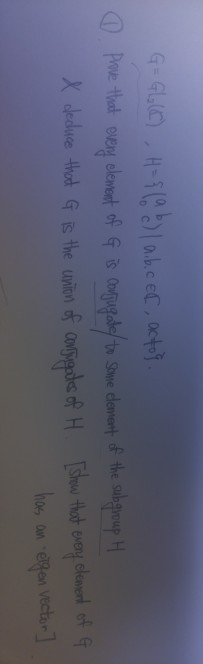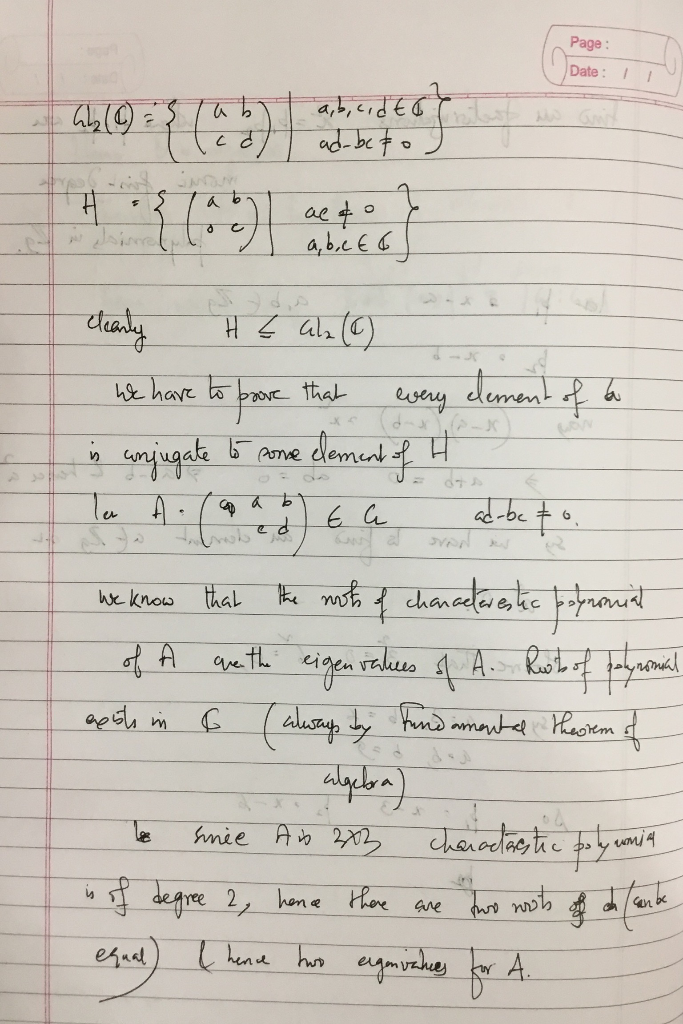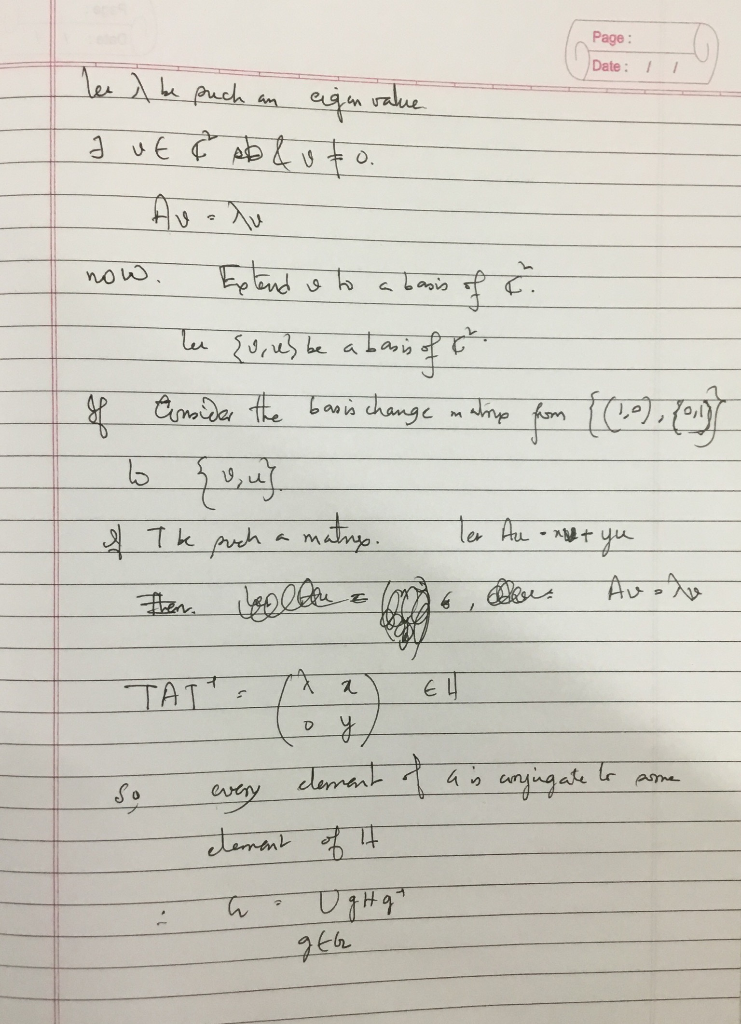Homework Help Question & Answers

# This problem is about abstract algebra, especially is group theory. Let G=GL2(C). which means gen...this problem is about abstract algebra, especially is group theory.

Let G=GL2(C). which means general linear group with each components are complex number.

and let H = {2x2 matrix (a b ; c d) l a,b,c are in Complex number, ac is not zero}

Prove that every element of G is conjugate to some element of the subgroup H and deduce that G is the union of conjugates of H [ Show that every element of GL2(C) has an eigenvector ]

o C ⓘ.pae tht eer elenent off scorreyto Swe denet of thes broupH 06 n e

#### Homework Answers

ReportAnswer #1Add Homework Help Answer
##### Add Answer of: This problem is about abstract algebra, especially is group theory. Let G=GL2(C). which means gen...
Your Answer: Your Name: What's your source?
Not the answer you're looking for? Ask your own homework help question. Our experts will answer your question WITHIN MINUTES for Free.
More Homework Help Questions Additional questions in this topic.

• #### 3. Let M be a manifold and let G C Homeo(M) be a group acting on M. Suppose that this group actio...

Need Online Homework Help?

Get FREE EXPERT Answers
WITHIN MINUTES
Related Questions